# MCQs on Power Systems

##### Page 17 of 67. Go to page 1 2 3 4 5 6 7 8 9 10 11 12 13 14 15 16 17 18 19 20 21 22 23 24 25 26 27 28 29 30 31 32 33 34 35 36 37 38 39 40 41 42 43 44 45 46 47 48 49 50 51 52 53 54 55 56 57 58 59 60 61 62 63 64 65 66 67
01․ Consider a three phase, 50 Hz, 11 kV distribution system. Each of the conductors is suspended by an insulator string having two identical porcelain insulators. The self capacitance of the insulators is 5 times the shunt capacitance between the link and ground. Find the voltage across the two insulators V1 and V2? (V1 = Voltage of disc nearest to the conductor)
V1 = 5 kV and V2 = 6 kV
V1 = 3.46 kV and V2 = 2.89 kV
V1 = 2.89 kV and V2 = 3.46 kV
V1 = 6 kV and V2 = 5 kV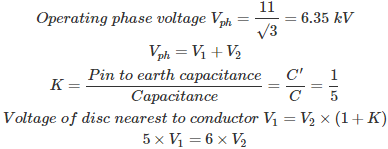By solving the above equations, V1 = 3.46 kV and V2 = 2.89 kV

02․ A string insulator has 4 units. The voltage across the bottom most unit is 33.33% of total voltage. Its string efficiency is
25%
33.33%
66.66%
75%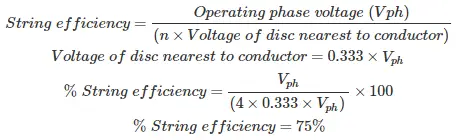Where, n = number of discs Operating phase voltage = Vph

03․ A string type insulator has three units with self capacitance C and ground capacitance of 0.2 C having a string efficiency of
78%
80%
82%
76%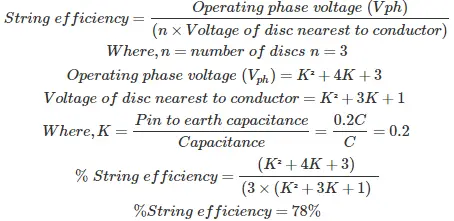04․ A guard ring is used in string type insulator having 3 units to improve string efficiency. Find the capacitance of metal link 2 if pin to earth capacitance of each unit is 1 F?
1 F
2 F
4 F
0.5 F

P = Metal link number = 2 n = Total number of discs = 3 C = Pin to earth capacitance of each unit = 1 µF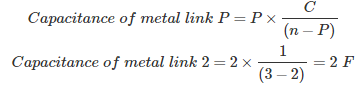05․ The surge impedance of a 400 km long overhead transmission line is 420 Ω. For a 200 km length of the same line, the surge impedance will be
210 Ω
400 Ω
840 Ω
420 Ω

Characteristic impedance (Zc): It will give the relation between voltage and current flowing through the transmission line which are depending on the transmission line parameters, i.e., R,G,L and C.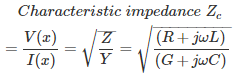For loss-less line. R = G = 0 Surge impedance Zs = √(L/C) Considering the length of the transmission line Zs = √(L×l/C×l) = √(L/C) Where, L = Inductance of line C = Capacitance of line l = Length of the line Therefore, surge impedance is independent of transmission line length and it depends only on inductance of the line and capacitance of the line.

06․ Which of the following represents the sag(S) of a transmission line?
S = wl²/8T
S = wl²/4T
S = wl²/2T
S = wl²/16T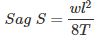Where, l = length of the conductor w = weight of the conductor T = tension

07․ The insulation resistance of the 20 km long underground cable is 8 MΩ. Other things being same, the insulation resistance of 10 km long cable will be
8 MΩ
4 MΩ
16 MΩ
32 MΩ

Conductor resistance Rc = ρL/A Rc ∝ L Where, ρ = Resistivity L = Length of the conductor A = Area of the conductor Insulation resistance Ri ∝ 1/L Therefore,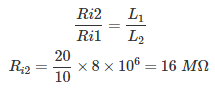08․ A 3-phase, 110 kV coaxial XLPE insulated power cable delivering power at 50 Hz, has a capacitance of 125 nF/km. If the dielectric loss tangent of XLPE is 2*10^-4, then dielcetric power loss in this cable in W/km/phase is
23.9
38.6
35.45
31.7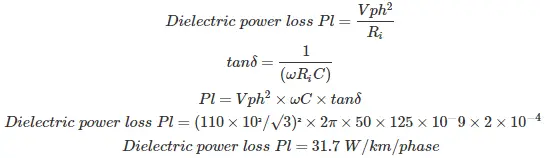tanδ = dielectric loss tangent Vph = phase voltage C = capacitance

09․ A cable has the following characteristics, L = 0.201 µH/m and C = 196.2 pF/m. The velocity of wave propagation through cable is
32 m/s
159.24 m/s
0.0312 m/s
159.24 m/µs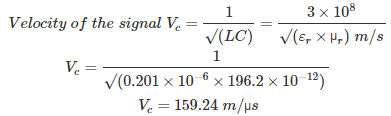Where, L = inductance of the line C = capacitance of the line

10․ Bonding is done at the both ends of the cable to reduce the
sheath loss
copper loss
iron loss
none of the above

In case of AC power cables due to the magnetic flux produced by the currents, induces voltages on sheath materials between the adjacent cable sheaths and due to this potential difference arcing will be developed which causes damages to the sheath. To reduce sheath losses and internal inductances and increase the resistance of the cable, bonding is done at both ends of the cable. Along with the bonding transposition is also used in 3-phase cable for reducing sheath losses and maintain uniform voltage.

<<<1516171819>>>